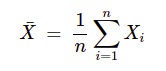Initially for MINDS(IITD) or CMINDS(IITB) there was a written test, if you qualify then you will proceed to the interview round.

You can follow the link given below for the syllabus. As both the written tests (no -ve marks) was more or less the same:

https://bit.ly/3Or8Nrw (IITB CMINDS)

In IITD there were 3 coding questions in addition to MCQs & MSQs and the test was conducted on HackerEarth platform.

IITD Interview Questions and Resources:

Around 40 people were shortlisted for the interview.The interview was conducted on 3rd June 2022(Till that time 3 coap rounds were over).My interview was scheduled at 4pm on Microsoft Teams.In my panel there were 3 professors.Each professor asked me questions from different subjects(given below).And the interview lasted for around 40-50 mins.

General Questions:

2. Currently what offers do you have?If you get M.Tech will you consider it or stick to MS(R) program and Why?

3. What are your favorite subjects? (Best answer, Linear Algebra & Probability for AI and Data science profile interviews.)

Also go through basic AI/ML stuff. Resource: https://bit.ly/3xy4tzO

Linear Algebra:

Take an equation Ax=B

1. Explain the solution of the system of linear equations w.r.t column space?

2. When will the system have a solution?

3. If matrix A has full rank, then for what B vectors does the system have a solution?

4. If the B vector doesn’t lie on the column space of A then how to get an approximate solution(projection concept)?

Resources:

https://bit.ly/3Qza41C (Must read upto 23rd video)

https://bit.ly/3xIokMP (Alternative to above resource)

Calculus:

1. What is a convex function?(Read thoroughly about concave & convex functions).

2. What is gradient?Give its geometrical significance.

3. What's a directional derivative?

Resources:

https://bit.ly/3zOvvpc

Probability & Statistics:

1. What is covariance for 2 random variables x & y?

2. Proof that for independent random variables covariance is 0.

3. Prove E(x+y)=E(x)+(y) for both independent & dependent cases.

4. Prove E(xy)=E(x)E(y) for an independent case.

5. A coin is tossed n times we define a random variable as follows,where xi is ith coin toss, prob. of head in each toss is p(success):

Find E(x(bar)) and Var(x(bar)).(Use linearity of expectation concept)Also do read thoroughly joint pdf,pmf, conditional expectation,total expectation,CDF etc.

Resources & Tips:

Keep handy all the distributions equations and graphs,specially normal distribution and approximation of poisson & binomial distributions using normal distribution.

Also go through proof of the memoryless property of geometrical & exponential distribution.

Recommended videos:

https://bit.ly/3OtYFhK (Must read upto 98th video)

https://bit.ly/3OoqmbC (Sampling distribution concept)

https://bit.ly/3n1d499

https://bit.ly/3OaaZ6O

https://bit.ly/3HBPE3G (Just go through week 3 important set of problems)

Algorithm:

1. Suppose you need to climb a stair of n steps and you can take at a time 1 step or extend your leg and take 2 steps or 3 steps at a time.Finds number of ways you can climb n steps.

(Hint: Take sol. as T(n).For T(n) we have 3 tree branches T(n-1)(taking 1 step at a time)/T(n-2) (taking 2 steps at a time)/T(n-3) (taking 3 steps at time) then again solve the subproblems of size (n-1)/(n-2)/(n-3) )

Verdict: Selected (IITD)

Note:

1. If you have a 650+ Gate score(Gen) you should definitely prepare well ahead as you will most probably get a call.Applicable for all branches.

2. IITB focuses more on LA & Prob.(specially joint distribution which was not part of the GATE syllabus).You have to study beyond the GATE syllabus.

3. You should never go out of studies after GATE for a long time else it will be difficult for you to crack the interviews.

4. Must be prepared with the B.Tech final year project.

Best of Luck for your selection :)

8
Like0
Love0
Haha0
Wow0
Angry0
Sad1
did you get IITD MINDS?

YesWas there interview for mtech as well?

Hi! @AnkitMazumder14 bhaiya,
Is python allowed in MINDS coding test?

1
Yes